# Queuing systems

Jump to: navigation, search

A common process in computing systems (and web servers) is managing a queue of requests. Typical queues can either by first-in, first-out (FIFO) or last-in, first-out (LIFO). The most common for web servers is the FIFO queue.

## Modeling

A schematic diagram of a simple queue is shown to the right. Messages arrive at rate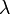and are stored in a queue. Messages are processed and removed from the queue at rate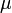. The average size of the queue is given by. There can be large variations in arrival rates and service rates, and the queue length builds up when the arrival rate is larger than the service rate. When the queue becomes too large, service is denied using an admission control policy.

The system can be modeled in many different ways. One way is to model each incoming request, which leads to an event-based model where the state is an integer that represents the queue length. The queue changes when a request arrives or a request is serviced. A discrete-time model that captures these dynamics is given by the difference equation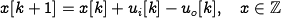whereand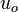are random variables representing incoming and outgoing requests on the queue. These variables take the values 0 or 1 with some probability at each time instant. In many cases it is possible to determine statistics of quantities like queue length and service time, but the computations can be quite complicated. To capture the statistics of the arrival and servicing of messages, we model each of these as a {\em Poisson process} in which the number of events occurring in a fixed time has a given rate, with the specific timing of events independent of the time since the last event. (The details of random processes are beyond the scope of this text, but can be found in standard texts such as [Pit99].)

A significant simplification can be obtained by using a flow model. Instead of keeping track of each request we instead view service and requests as flows, similar to what is done when replacing molecules by a continuum when analyzing fluids. Assuming that the average queue lengthis a continuous variable and that arrivals and services are flows with ratesand, the system can be modeled by the first-order differential equationwhereis the maximum service rate and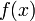is a number between 0 and 1 that describes the effective service rate as a function of the queue length.

It is natural to assume that the effective service rate depends on the queue length because larger queues require more resources. In steady state we have, and we assume that the queue length goes to zero whengoes to zero and that it goes to infinity whengoes to 1. This implies thatand that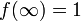. In addition, if we assume that the effective service rate deteriorates monotonically with queue length, then the functionis monotone and concave. A simple function that satisfies the basic requirements is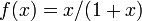, which gives the model (FAQ)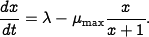This model was proposed by Agnew~\cite{Agnew76}. It can be shown that if arrival and service processes are Poisson processes, the average queue length is given by equation~\eqref{eq:webserv:webseverdynamics} and that equation~\eqref{eq:webserv:webseverdynamics} is a good approximation even for short queue lengths; see Tipper~\cite{Tip+Sun90}.

To explore the properties of the model we will first investigate the equilibrium value of the queue length when the arrival rateis constant. Setting the derivative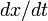to zero in the dynamics and solving for, we find that the queue lengthapproaches the steady-state valueThe figure to the right shows the steady-state queue length as a function of, the effective service rate excess. Notice that the queue length increases rapidly asapproaches. To have a queue length less than 20 requires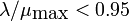. The average time to service a request is, and it increases dramatically asapproaches.

The next figure illustrates the behavior of the server in a typical overload situation. The solid line shows a realization of an event-based simulation, and the dashed line shows the behavior of the flow model. The maximum service rate is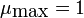, and the arrival rate starts at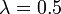. The arrival rate is increased to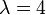at time 20, and it returns toat time 25. The figure shows that the queue builds up quickly and clears very slowly. Since the response time is proportional to queue length, it means that the quality of service is poor for a long period after an overload. This behavior is called the rush-hour effect and has been observed in web servers and many other queuing systems such as automobile traffic.

The dashed line in th figure shows the behavior of the flow model, which describes the average queue length. The simple model captures behavior qualitatively, but there are variations from sample to sample when the queue length is short. It follows from the model that the rate of change is approximately 3 messages/second when the queue length builds up at time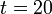, and approximately 0.5 messages/second when the queue length decreases after the build up. The time to return to normal is thus approximately 6 times the overload time.